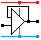Home

rOm
Quest
Glossary

Random
Page
Search
Site
Lush
Sim
Class
Subject
Images

Help
FAQ
Sign
Up
Log
In
 A Level     AC Circuits     Active Filters     >Passive Filters<     Tuned Circuits

# AC Circuits Passive Filters

Site for Eduqas/WJEC - Go to the AQA site.

A Level    Filters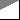Questions 0 to 38   -->  View All

Filters are used to pass or block selected ranges of frequencies.

• Sub-woofer: this uses a low pass filter to send the bass to the sub-woofer. The mid range and high frequencies are removed.
• Tweeter: this uses a high pass filter to send the treble audio to the tweeter. The mid range and bass are removed.
• Low Pass Filters are important when converting analogue signals to digital. If frequencies greater than half the sampling rate get to the converter, unwanted and unpleasant alias signals appear at the output.

## Filter Breakpoint Frequency

At the breakpoint frequency of a filter ...

• the output voltage drops to 0.7 of the maximum output voltage.
• the output voltage power to 0.5 of the maximum output power.
• the reactance of the capacitor is equal to the resistance of the resistor.

## Passive RC Filters

• In the graphs below, the blue vertical lines show the breakpoint frequencies.
• Both the X and Y graph axes are log scales.
• Passive filters attenuate the signals. If this is a problem, active filters should be used instead.

### High Pass - Bass Cut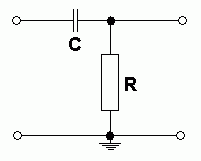• The capacitor blocks DC and low frequencies.
• The capacitor passes high frequencies easily.

### Low Pass - Treble Cut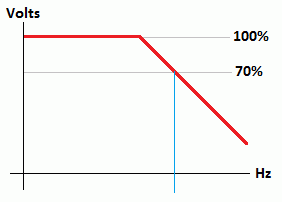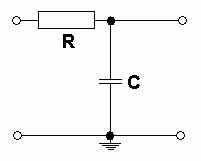• The capacitor has no effect on DC or low frequencies.
• The capacitor decouples high frequencies to ground.

### Band Pass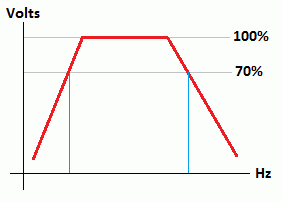• This circuit is a combination of
high and low pass circuits

### Capacitor Reactance

• Reactance is the Alternating Current equivalent of resistance.
• It is measured in Ohms.
• The formula:    Xc   =   1 / (2 π f C)

### Breakpoint Frequency Calculations

• These calculations apply to both passive and active filters.
• At the breakpoint frequency, the reactance of the capacitor equals the resistance of the resistor.

• R = Xc

• Xc = 1 / (2 π f C)

• R = 1 / (2 π f C)

• R f = 1 / (2 π C)

• f = 1 / (2 π R C)

• C = 1 / (2 π R f)

### Impedance Calculations

The formula below is identical to the Pythagoras triangle formula. So ancient Greeks were prescient about filter impedances!

Z2 = ( R2 + X2 )        and        Z = √( R2 + X2 )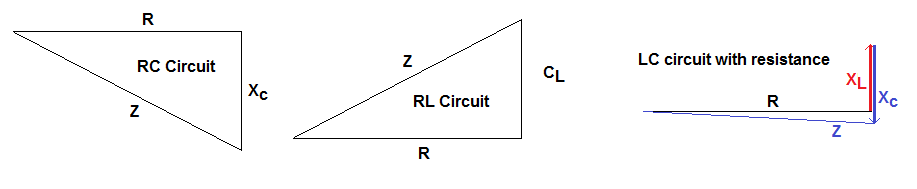Subject Name     Level     Topic Name     Question Heading     First Name Last Name Class ID     User ID

 CloseEscapeX Q: qNum of last_q     Q ID: Question ID         Score: num correct/num attempts         Date Done

Question Text

image url

Help Text
Debug

• You can attempt a question as many times as you like.
• If you are logged in, your first attempt, each day, is logged.
• To improve your scores, come back on future days, log in and re-do the questions that caused you problems.
• In the grade book, you can delete your answers for a topic before re-doing the questions. Avoid deleting unless you intend re-doing the questions very soon.